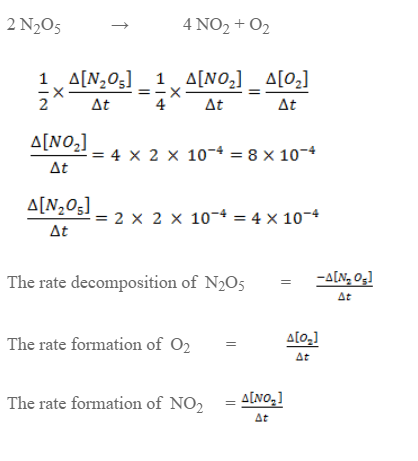# Rate Of Reaction And Stoichiometric Coefficient

Let’s consider a reaction

N2 + 3H2 → 2NH3

The rate of reaction can be expressed as,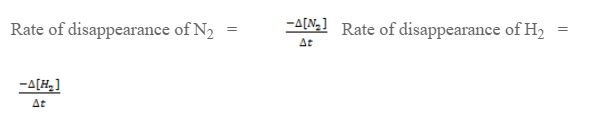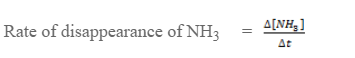Here, during disappearance of 1 mole of N, 3 mols of H2 are disappeared. So, the rate of disappearance of H2 is 3 times the rate of disappearance of N2.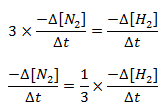The disappearance of 1 mole of N2 gives 2 moles of NH3. So the rate of formation of NH3 is 2 times the rate of disappearance.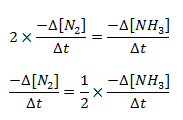The equivalent rate of reaction is,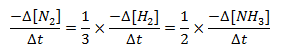Therefore, the equivalent rate of a reaction is obtained by dividing the rate of each species by its stoichiometric coefficient,

aA + bB → cC + dD

The equivalent rate of reaction is,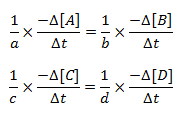For the reaction,
H2 + I2 → 2HI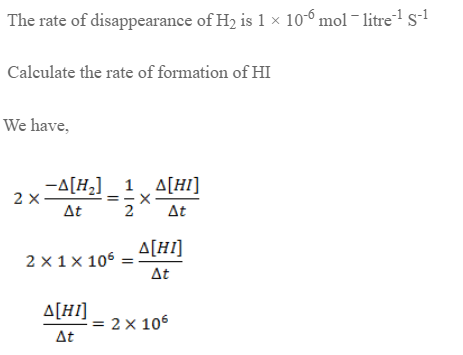Express the rate of reaction in terms of all species for decomposition of N2O5. If the rate of formation of O2 is 2× 10-4 mol lit-1 S-1 calculate the rate of disappearance of N2O5 and rate of formation of N2.